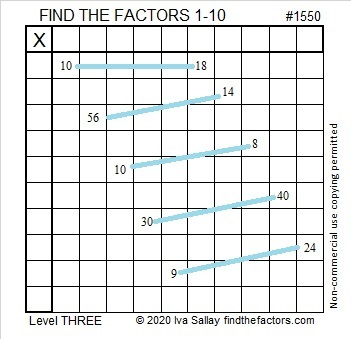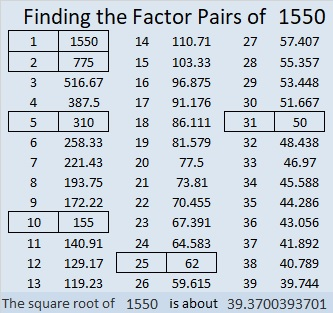# 1550 Lucky for You: Solving This Puzzle Is as Easy as Climbing Down a Ladder

Contents

### Today’s Puzzle:

It’s Friday the 13th, so don’t walk under any ladders! Still, there isn’t any reason to avoid them entirely. Because this ladder puzzle is a level 3, the clues are given in a logical order to help you find the solution. Start at the top of the ladder, find the common factor of 10 and 18, then work your way down the ladder rung by rung, writing all the numbers from 1 to 10 in both the first column and the top row until you reach the bottom of the ladder.  Good Luck!Here’s the same puzzle with no colors to distract you.### Factors of 1550:

• 1550 is a composite number.
• Prime factorization: 1550 = 2 × 5 × 5 × 31, which can be written 1550 = 2 × 5² × 31.
• 1550 has at least one exponent greater than 1 in its prime factorization so √1550 can be simplified. Taking the factor pair from the factor pair table below with the largest square number factor, we get √1550 = (√25)(√62) = 5√62.
• The exponents in the prime factorization are 1, 2, and 1. Adding one to each exponent and multiplying we get (1 + 1)(2 + 1)(1 + 1) = 2 × 3 × 2 = 12. Therefore 1550 has exactly 12 factors.
• The factors of 1550 are outlined with their factor pair partners in the graphic below.### More about the Number 1550:

1550 is the hypotenuse of TWO Pythagorean triples:
434-1488-1550, which is (7-24-25) times 62, and
930-1240-1550, which is (3-4-5) times 310.

This site uses Akismet to reduce spam. Learn how your comment data is processed.